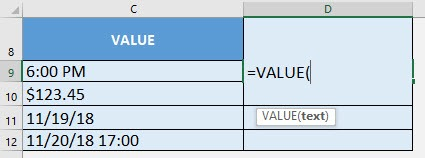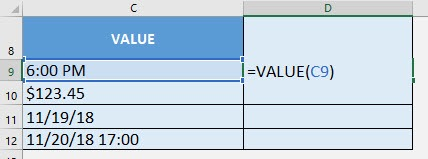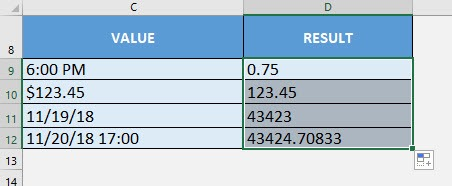What does it do?

Converts text into a numeric value

Formula breakdown:

=VALUE(text)

What it means:

=VALUE(text to be converted to a numeric value)

We can easily change text into its corresponding numeric value using the VALUE formula in Excel. It is also interesting as we can try out different types and see how it looks like in its numeric format.

Let us try out a couple of data types: Dates, currency, and time to name a few!

I explain how you can do this below:STEP 1: We need to enter the VALUE function in a blank cell:

## =VALUE(STEP 2: The VALUE arguments:

## text

What is the text to be converted to a numeric value?

Select the cell containing the text that you want to convert:

## =VALUE(C9)Apply the same formula to the rest of the cells by dragging the lower right corner downwards.You can see that the text are all now in their numeric values!How to Use the VALUE Formula in Excel